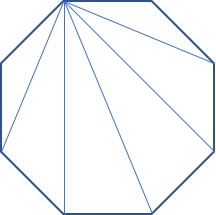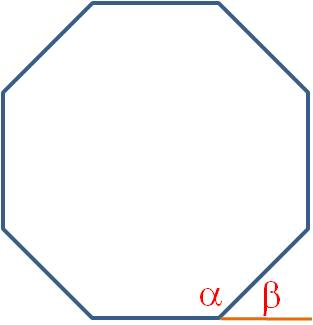## finding the number of diagonals in a polygon

How many diagonals are there in an octagon?Instead of trying to count them all, use the formula for the number of diagonals in any polygon:$d= \dfrac{n(n-3)}{2}$

where n = the number of sides and d= the number of diagonals. For an octagon, n= 8, so$d= \dfrac{8(8-3)}{2} = 20$

This is a good formula to memorize and its derivation is pretty simple, so let’s see it. We will use the same octagon diagram, but only draw the diagonals from one vertex:You can see that from this vertex on the upper left, a diagonal can be drawn to every vertex except the two adjacent vertices and to itself. So the number of diagonals from any vertex in an n-gon is (n-3). Since there are n vertices, the total number of diagonals is n(n-3). But we’ve just counted every diagonal twice (once from each endpoint), so to find the number of different diagonals, we divide by two. This gives us the formula above.

## finding internal or external angles of regular polygons

How do you find the measure of an internal angle or an external angle on a regular polygon? It’s quite easy, if you memorize two formulas. All you have to do is count the number of sides on the polygon and plug it into the formula.

For internal angles, use the formula$\alpha = \dfrac{180(n-2)}{n}$

For external angles, the formula is even easier:$\beta = \dfrac{360}{n}$

For example, here is a regular octagon, so n = 8. The internal angle is labeled α and the external angle is β.$\alpha = \dfrac{180(8-2)}{8}=135 \textdegree$$\beta = \dfrac{360}{8} = 45 \textdegree$

And here’s a trick to save you some time: You can see in the figure that the internal and external angles are always supplementary. (This is true in any polygon, even if it isn’t regular.) So if you need to find the internal angle, you can find the external angle instead with the simpler formula. Then the supplement to that angle is the internal angle!

Blue Taste Theme created by Jabox